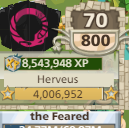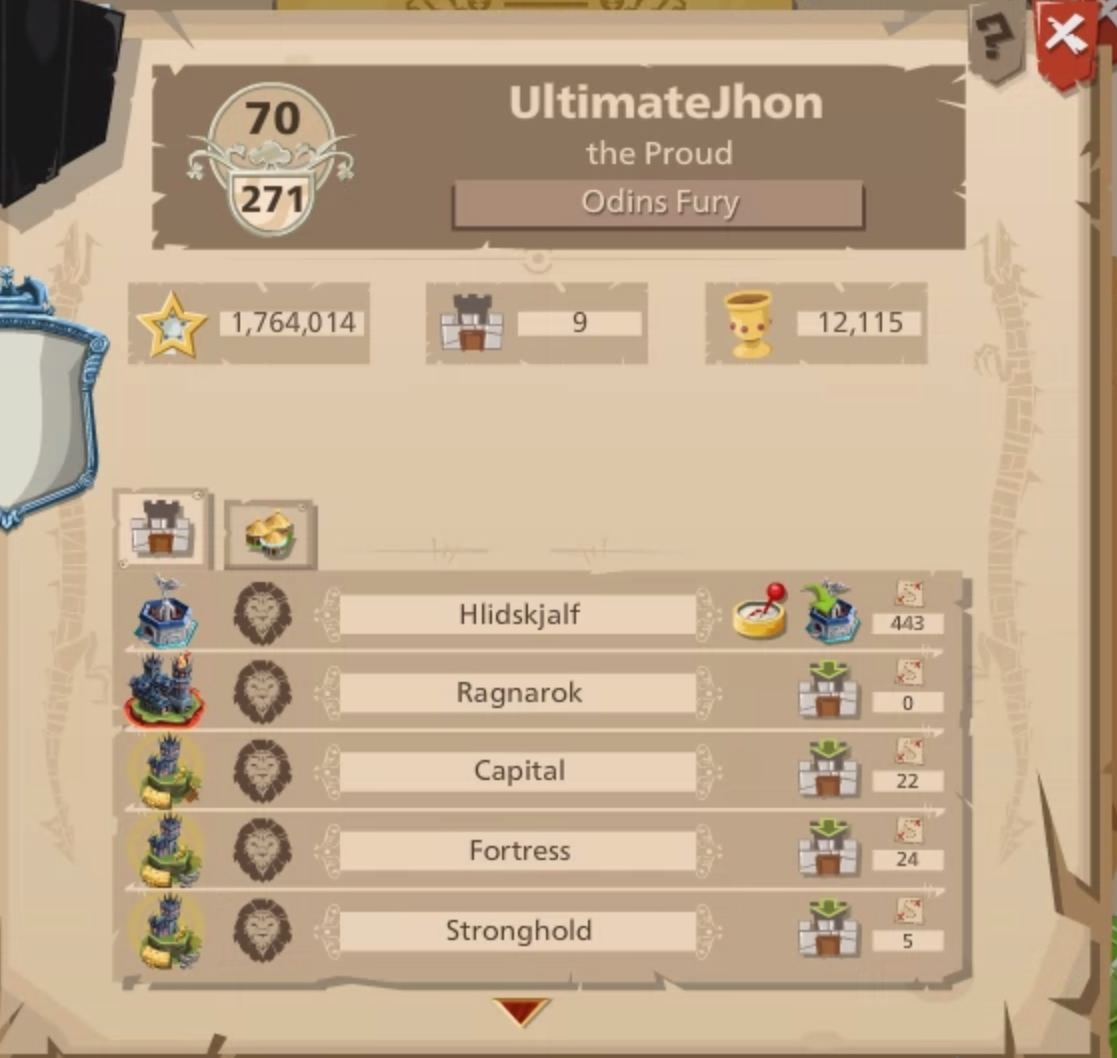# Highest po on a colossus

What is the highest po on a colossus ever seen?

• Let me do the math some german guy spend 2 billion coins on one meaning
361po• 550
#TheDreamLivesOn• 6,000

Retired Recruiter of BattleHorn• DiamondBee has a 550 one
Recruiter @ The Black Flag• edited 03.09.2017
69

Edit: anything higher than 69 is illuminati
• 550

LOL

how much resources does that cost thats insane

• how much do you have in resources or coins? the more you put in the higher the po
Marty Maurder @ en 1
• If the formula I have is correct it required
PO=26*ln(Points)-78
so that means Points=10^((PO+78)/26)
Po=550
so we have
10^((550+78)/26)
10^((628)/26)
10^(24,15384615384615)
=
1.4251027e+24
=1425102670151547945000000
as minimum points
and as we know 50 Wood = 1 point or 34 Stone = 1 point
assuming was all paid in wood it was
71255133507577397250000000 wood
or
48453490785152630130000000 stone
if 50/50 we have an average of 42 per point so we have a total of
59854312146365013690000000
or
712551335075773972500000 wood and 712551335075773972500000 stone
in coins it is 92 per point so I'd have been
131109445653942410940000000
but this is impossible as 2200000000 is the limit in coins you can have
so now what if you had the maximum amount of skips and bought during event ~30k so let's say you used all the those on nomads and have all loot bonus and all loot bonuses and then let's say you loot 81k wood 81k stone n food then you'd end up with
30.000*81.000.2=4860000000 in total which is
4860000000/59854312146365013690000000=0.0000000000000000812 or otherwise said 0.00000000000000812% of what you need for a 550 po colossus
now let's say multiple people loot that much how many would you need?
1/0.0000000000000000812=12315270935960591.1330049261083743842365 so around 12315270935960591 people doing that so nope now with coin's let's say you loot 1000000  per attack using the ruby pigs all 4 waves you'd end up with
1000000*30000=30000000000 which is over cap 13 times but if we ignore that and say he spends it all fast enough we still only have
30000000000 /131109445653942410940000000=0.0000000000000002288 or 0.00000000000002288% which yes is closer than the ressource one but even if everyone on the us server send her 350 coin gifts (assuming a 7 day colossus and 50 gifts [max] each day) I'd not be enough.
And while my formula for po to points is not 100% excact it gives and estimate and even if points where lower by a fe1 10^-n I'd still not be realistic to get a 550 po colossus. Thus I'd say that no one has a 550 po colossus.

think u spent waaaaaaaay too much time on that lol
I love maths, I take part in maths competitions, that was like free training xD• If the formula I have is correct it required
PO=26*ln(Points)-78
so that means Points=10^((PO+78)/26)
Po=550
so we have
10^((550+78)/26)
10^((628)/26)
10^(24,15384615384615)
=
1.4251027e+24
=1425102670151547945000000
as minimum points
and as we know 50 Wood = 1 point or 34 Stone = 1 point
assuming was all paid in wood it was
71255133507577397250000000 wood
or
48453490785152630130000000 stone
if 50/50 we have an average of 42 per point so we have a total of
59854312146365013690000000
or
712551335075773972500000 wood and 712551335075773972500000 stone
in coins it is 92 per point so I'd have been
131109445653942410940000000
but this is impossible as 2200000000 is the limit in coins you can have
so now what if you had the maximum amount of skips and bought during event ~30k so let's say you used all the those on nomads and have all loot bonus and all loot bonuses and then let's say you loot 81k wood 81k stone n food then you'd end up with
30.000*81.000.2=4860000000 in total which is
4860000000/59854312146365013690000000=0.0000000000000000812 or otherwise said 0.00000000000000812% of what you need for a 550 po colossus
now let's say multiple people loot that much how many would you need?
1/0.0000000000000000812=12315270935960591.1330049261083743842365 so around 12315270935960591 people doing that so nope now with coin's let's say you loot 1000000  per attack using the ruby pigs all 4 waves you'd end up with
1000000*30000=30000000000 which is over cap 13 times but if we ignore that and say he spends it all fast enough we still only have
30000000000 /131109445653942410940000000=0.0000000000000002288 or 0.00000000000002288% which yes is closer than the ressource one but even if everyone on the us server send her 350 coin gifts (assuming a 7 day colossus and 50 gifts [max] each day) I'd not be enough.
And while my formula for po to points is not 100% excact it gives and estimate and even if points where lower by a fe1 10^-n I'd still not be realistic to get a 550 po colossus. Thus I'd say that no one has a 550 po colossus.

think u spent waaaaaaaay too much time on that lol
I love maths, I take part in maths competitions, that was like free training xD
Are you bullied in school?
diaper boy @ USA
onion god @ AU
• If the formula I have is correct it required
PO=26*ln(Points)-78
so that means Points=10^((PO+78)/26)
Po=550
so we have
10^((550+78)/26)
10^((628)/26)
10^(24,15384615384615)
=
1.4251027e+24
=1425102670151547945000000
as minimum points
and as we know 50 Wood = 1 point or 34 Stone = 1 point
assuming was all paid in wood it was
71255133507577397250000000 wood
or
48453490785152630130000000 stone
if 50/50 we have an average of 42 per point so we have a total of
59854312146365013690000000
or
712551335075773972500000 wood and 712551335075773972500000 stone
in coins it is 92 per point so I'd have been
131109445653942410940000000
but this is impossible as 2200000000 is the limit in coins you can have
so now what if you had the maximum amount of skips and bought during event ~30k so let's say you used all the those on nomads and have all loot bonus and all loot bonuses and then let's say you loot 81k wood 81k stone n food then you'd end up with
30.000*81.000.2=4860000000 in total which is
4860000000/59854312146365013690000000=0.0000000000000000812 or otherwise said 0.00000000000000812% of what you need for a 550 po colossus
now let's say multiple people loot that much how many would you need?
1/0.0000000000000000812=12315270935960591.1330049261083743842365 so around 12315270935960591 people doing that so nope now with coin's let's say you loot 1000000  per attack using the ruby pigs all 4 waves you'd end up with
1000000*30000=30000000000 which is over cap 13 times but if we ignore that and say he spends it all fast enough we still only have
30000000000 /131109445653942410940000000=0.0000000000000002288 or 0.00000000000002288% which yes is closer than the ressource one but even if everyone on the us server send her 350 coin gifts (assuming a 7 day colossus and 50 gifts [max] each day) I'd not be enough.
And while my formula for po to points is not 100% excact it gives and estimate and even if points where lower by a fe1 10^-n I'd still not be realistic to get a 550 po colossus. Thus I'd say that no one has a 550 po colossus.

think u spent waaaaaaaay too much time on that lol
I love maths, I take part in maths competitions, that was like free training xD
Are you bullied in school?
So what if he was?

When he leaves school and gets employed and paid more than the bullies, it wont matter.WAR PLAYER
• Not sure about coin collossus, but for resource collossus I thought the formula is:
Point = 20 x 10^(PO/60).

• If the formula I have is correct it required
PO=26*ln(Points)-78
so that means Points=10^((PO+78)/26)
Po=550
so we have
10^((550+78)/26)
10^((628)/26)
10^(24,15384615384615)
=
1.4251027e+24
=1425102670151547945000000
as minimum points
and as we know 50 Wood = 1 point or 34 Stone = 1 point
assuming was all paid in wood it was
71255133507577397250000000 wood
or
48453490785152630130000000 stone
if 50/50 we have an average of 42 per point so we have a total of
59854312146365013690000000
or
712551335075773972500000 wood and 712551335075773972500000 stone
in coins it is 92 per point so I'd have been
131109445653942410940000000
but this is impossible as 2200000000 is the limit in coins you can have
so now what if you had the maximum amount of skips and bought during event ~30k so let's say you used all the those on nomads and have all loot bonus and all loot bonuses and then let's say you loot 81k wood 81k stone n food then you'd end up with
30.000*81.000.2=4860000000 in total which is
4860000000/59854312146365013690000000=0.0000000000000000812 or otherwise said 0.00000000000000812% of what you need for a 550 po colossus
now let's say multiple people loot that much how many would you need?
1/0.0000000000000000812=12315270935960591.1330049261083743842365 so around 12315270935960591 people doing that so nope now with coin's let's say you loot 1000000  per attack using the ruby pigs all 4 waves you'd end up with
1000000*30000=30000000000 which is over cap 13 times but if we ignore that and say he spends it all fast enough we still only have
30000000000 /131109445653942410940000000=0.0000000000000002288 or 0.00000000000002288% which yes is closer than the ressource one but even if everyone on the us server send her 350 coin gifts (assuming a 7 day colossus and 50 gifts [max] each day) I'd not be enough.
And while my formula for po to points is not 100% excact it gives and estimate and even if points where lower by a fe1 10^-n I'd still not be realistic to get a 550 po colossus. Thus I'd say that no one has a 550 po colossus.

think u spent waaaaaaaay too much time on that lol
I love maths, I take part in maths competitions, that was like free training xD
Are you bullied in school?
So what if he was?

When he leaves school and gets employed and paid more than the bullies, it wont matter.
Ok. It was a joke though. So don't go all SJW on me. lol
diaper boy @ USA
onion god @ AU